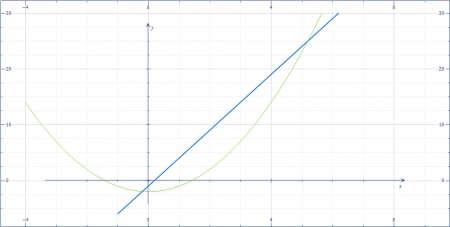# Use a graphing utility with the specified viewing window to find the points of intersection of...

## Question:

Use a graphing utility with the specified viewing window to find the points of intersection of the graphs of the following functions.

{eq}f(x) = 5x - 1, g(x) = x^2 - 2; [-4, 9]\; \text{by}\; [-6, 30] {/eq}

Select the correct choice below and, if necessary, fill in the answer box to complete your choice.

A. The intersections are _____.

(Type an ordered pair using integers or decimals. Round to the nearest hundredth as needed. Use a comma to separate answers as needed.)

B. There are infinitely many solutions of the form (x, _____), where x represents all the real numbers (Type an expression using x as the variable.)

C. There are no points of intersection.

## Graphing

Graphing a function is very useful. As can be seen here, it helps us to see where the functions intersect. This also helps us in finding an initial guess when working towards finding a more accurate solution.

The two functions have been graphed below.As can be seen, there are two intersection points within the specified viewing window. These are {eq}x=-0.19 {/eq} and {eq}x=5.19 {/eq}.

The corresponding y-coordinates are approximately {eq}y=-1.96 {/eq} and {eq}y=24.95 {/eq}.

Thus, the two points at which the two curves intersect are {eq}\left [-0.19,-1.96 \right ] {/eq} and {eq}\left [5.19,24.95\right ] {/eq}